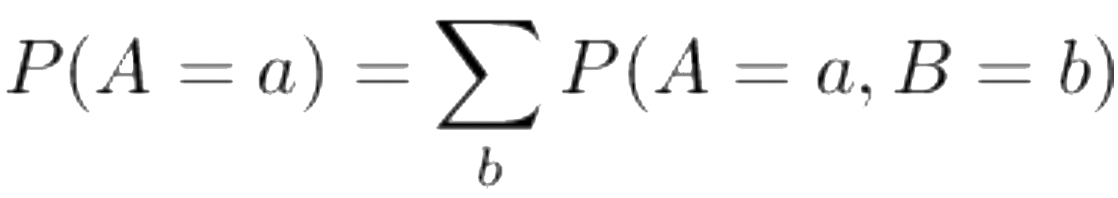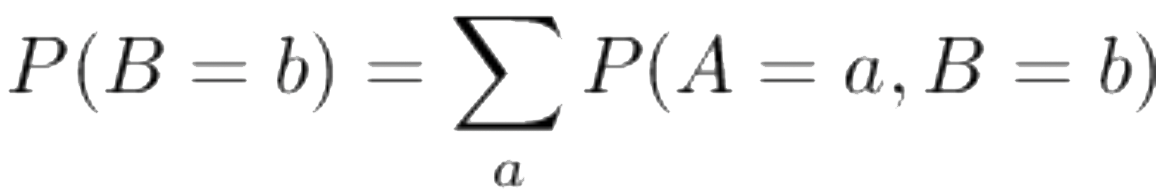##### April 20, 2020

Human society has a lot of very, very hard decisions to make in the days ahead. These will require us to make a host of predictions: How will the epidemic spread if we do this versus that? How will the economy be affected if we follow that course of action rather than this one?

One of the major challenges in making these predictions is that they require us to specify the dynamic processes involved. Some of the models one can use to do this are based on equations, which are (typically) then approximated on a computer. Some models are instead based on massive simulations called “agent-based models,” which were pioneered, in large part, at the Santa Fe Institute.

Whatever model we use to predict the future, we have to specify the initial condition of the variables in those models. We need to specify the current state of affairs, quantified with numbers ranging from the value of R0 for the SARS-CoV-2 virus, to how many people have been furloughed rather than fired. In turn, to get those initial condition numbers, we need to convert some “noisy” data that we have  gathered into a probability distribution over the initial condition numbers.

To illustrate the great challenge that we face, I’m going to describe why even just finding the distribution over the initial condition numbers for our models — never mind using those models to make the excruciating choices that await us — is fraught, with no right or wrong answer.

Converting noisy data into a probability distribution is the subject of the field of statistics. Broadly speaking, there are two branches of  statistics, and they provide different guidance for how to form such a probability distribution. To understand the older (and recently resurgent) of the two, a little algebra helps.

Suppose we have two random variables, A and B. The probability that those variables take the values a and b simultaneously is P(A = a, B = b). What is the probability that A = a, no matter what value B has? This is called the “marginal distribution” of A, and if you think about it, it is just the sum of P(A = a, B = b) over all possible values b:.

Similarly, the marginal distribution for values of B is.

What is the probability that B will have the value b, given that A has the value a? If you (again) think about it a bit, this “conditional distribution” is just.

Just like the quadratic equation holds, just like the sum of any two odd numbers is an even number, just like the product of two odd numbers is an odd number, the equations above mean that.

The left-hand side of the equation is the same. And the denominator of the righthand side is the same. All I have done is substituted the joint distribution formula in the numerator of the right-hand side with an equivalent distribution — the probability of A given B for all values of B, which is just another way of writing the joint distribution.

This simple formula for converting a conditional distribution (A given B) into its “opposite” (B given A) is known as Bayes’ theorem.1 To illustrate it, suppose that there is a blood test for COVID-19 that ideally would say “+” if and only if one has the virus. Suppose it is 90 percent accurate, in the sense that the table for the conditional distribution P(test result | health status) is:

 + 0.9 0.1 - 0.1 0.9 Sick Well

This table can be summarized by saying that the false positive rate is 0.1 and the false negative rate is 0.1.

Suppose you get tested — and are positive. How scared should you be? According to P(test | health), you might think that there’s a 90 percent chance that you have the virus. But the truth is otherwise, and this is where the Bayes equation comes in.

Suppose that only 1 percent of the population is infected, so that — everything else being equal — the “prior” probability that you are sick, P(health = sick), is 1 percent. So, according to Bayes, the associated table for what you’re interested in, P(health status | test result) is (approximately)

 Sick 0.001 0.1 Well 0.999 0.9 - +

For example, P(well | +) / P(sick | +) = P(+ | well) x P(well) / P(+ | sick) x P(sick) = 11, so P(well | +) ~ 0.9. So there’s actually only a 10 percent chance that you’re sick — still not good, but certainly less frightening.

Bayes’ theorem has been elevated to the status of the scientific deities, as either the source of all truth and light, or of unending evil. Why?

Note that to apply Bayes’ theorem we needed to know the prior. And in the case of the COVID-19 pandemic this is one of those estimates that we do not have; we do not know how many people in the population are infected. That is not just true in the example of blood tests; it is also true when (for example) using current data to set the initial condition numbers for our models for predicting the future course of the pandemic. Where do we get that prior from? In the case of blood tests it was relatively simple. But in more complicated scenarios — like formulating the probability distribution of the initial condition numbers for our models of how the pandemic will unfold — it can be a very difficult question. Answering this question, and using our answers to calculate what we want to know, is called “Bayesian statistics.”

End of story? Not quite. Bayes’ theorem embodies one of the deepest truths of life: garbage in, garbage out. Adopt a stupid prior, and you get a stupid answer. Not surprisingly then, Bayesian statistics was badly misused in the past, and produced many horrible results. Frustration with these results led people to create the main competitor to Bayesian statistics, called “frequentist statistics.”

Can we justify frequentist techniques as actually being Bayesian, just for some implicit prior? If so, might frequentist techniques actually be a way to generate implicit priors, without violating the laws of math? Well, no. Even one of the most reliable, most widely used of frequentist statistics tools — the “bootstrap” — can be proven not to agree with a Bayesian analysis for any prior.2

This does not mean that we “should” use Bayesian statistics, in any normative sense, when we come up with the numbers to put into our models of the next year. (I myself am a great fan of the frequentist technique of bootstrap.) Even if rather than feeding garbage into Bayes’ theorem we feed it ambrosia, we will still be making an assumption. If the virus — if our global economy — doesn’t happen to agree with our Bayesian assumption, it does not matter whether our mathematics is correct. There is no free lunch.

David Wolpert
Santa Fe Institute

REFERENCES

1. Berger, James O. 2013. Statistical Decision Theory and Bayesian Analysis. Springer Science & Business Media.
2. Wolpert, D.H. 1996. “The Bootstrap is Inconsistent with Probability Theory,” in Maximum Entropy and Bayesian Methods 1995, K. Hanson and R. Silver, eds. Kluwer Academic Press.

T-019 (Wolpert) PDF